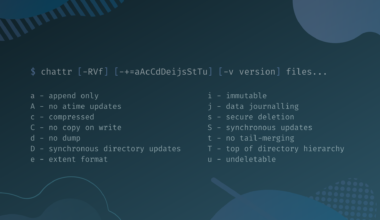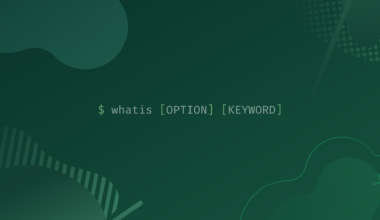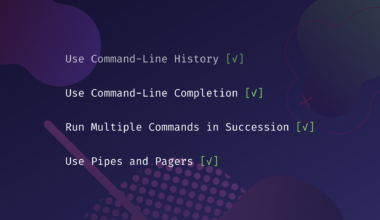# Bash While Loop

In almost all programming languages, a loop is an easy and basic method used to repeat single or multiple instructions more than one time.

For example, if you want to perform some arithmetic operation like adding two numbers or any other operation multiple times, you should use a loop. This saves you from writing the same instructions again and again.

The same concept can be applied in Bash scripting to execute commands that are needed repeatedly. There are three types of loops that you can use in Bash scripting, which are the for loop, the `while` loop, and the `until` loop.

In this tutorial, you will learn about the `while` loop, its syntax, and how to use a `while` loop in a Bash script.

All this is discussed in the sections that follow along with suitable examples.

## Bash While Loop

The while loop is usually used when you don’t know in advance how many times you want to execute a loop.

Does this mean that the while loop will keep on executing the instructions for an unknown number of times?

YES, this is the case, the while loop will keep on executing until a specified condition is met.

For instance, you ask the user to enter Y to exit the loop. In each iteration of the loop, the user will be prompted to enter their choice and the loop will keep on executing several times (which you cannot determine at the start of the loop).

An analogy is available in computer games, where you are asked to play again or not at the end of the game. In such scenarios, the programmer doesn’t know how many times a person will play the game.

This is in contrast to for loop, where you specify a final value for the loop to execute. Whereas in the case of while, you don’t know in advance how many times the loop will execute.

However, in simple cases like a positive number less than 10, you can count and guess how many times a while loop will execute.

## Syntax

The syntax of the while loop in a bash script is the following.

```while [CONDITION]
do
COMMANDS
done```

The loop starts with the while keyword, followed by a condition (usually written in square brackets).

The while loop is controlled by this condition. It means the loop will execute the commands repeatedly until the condition remains true.

Once the condition becomes false, it terminates the while loop. All the commands are enclosed between do and done keywords.

## Three Important Points To Remember

1. It is important to mention that you will need a loop variable to control the execution of the while loop. In the bash script, you can simply write the name of a variable and assign it a value using an equal sign in between (e.g., n=1). This value is called the initial value (which means the loop will start from this value). This variable has to be declared and initialized before starting the While loop.
2. To access the value contained in the variable (e.g., inside the condition of a `while` loop or in an echo command), you have to use a dollar sign (\$) before the variable name. An example is given below.
`echo "\$n"`
3. The loop variable should be incremented/decremented inside the body of the loop (i.e., between do and done keywords), otherwise, the loop would become infinite (means would execute forever).

## Explanation of while Loop Components

To explain the components of the while loop and how it works in the bash script, let’s explore some examples.

hello.sh
```#! /bin/bash
i=0
while [ \$i -lt 10 ]
do
echo "\$i"
i=\$(( i + 1 ))
done```

In the above example, a loop variable i is used and assigned an initial value of `0` in the first line. Inside the while loop, a while condition, otherwise, you will get an error.

The value of i (which is initially 0 and obtained via \$i) is compared with 10 using the `-lt` (less than) option. Another option that can be used here is `-le` (less than or equal to).

The first line in the body of the loop just prints the value of i using the echo command. Whereas the second line increments the value of i by `1`. This is achieved by using a \$ sign and double parenthesis. Remember to give spaces here too, else you will get an error.

After this line, the value of i is incremented by `1`. This value when checked with the condition in the next iteration is still less than `10`, so the loop will again iterate, as the condition is still true. In this way, the loop will continue executing until the condition becomes false (meaning when the value of i becomes greater or equal to `10`).

The output of the above While loop is shown below.

#### Example 1

`./hello.sh`
Output
```0
1
2
3
4
5
6
7
8
9```

Try to change the parameter `-lt` with `-le` and see how the output is changed.

#### Example 2

hello.sh
```#! /bin/bash
n=1

while [ \$n -le 10 ]
do
echo "Number :\$n"
((n++))
done```

In the first line, the loop variable is initialized by giving the value `1`. The condition in the while loop limits the value of n up to 10.

In other words, the loop will repeat the code till the value of n becomes `10`. The echo command will simply display the value of n. In the last line, you can see that the loop variable is incremented by `1` in the C++ style.

The resulting output will be numbers from 1 to 10 as shown below.

`./hello.sh`
Output
```Number: 1
Number: 2
Number: 3
Number: 4
Number: 5
Number: 6
Number: 7
Number: 8
Number: 9
Number: 10```

## Infinite while loop

When the while keyword is not followed by a condition (called null condition), it assumes that the condition is true. Therefore, the while loop will run forever until you press Ctrl + C or Ctrl + Z to forcibly stop the loop.

Let’s see an example of an infinite while loop.

hello.sh
```#! /bin/bash

while:
do
echo "hello world"
done
```

Here, while is used with null condition (or you can say without a condition). Consequently, the loop would run the echo command an infinite number of times.

You can also write `[ 1 ]` or `true` as a condition to make an infinite while loop (because `1` means true and while loop executes if the condition is true).

Since the condition in both of these cases will remain true forever, the loop will execute infinitely. The output of this loop is shown below.

`./hello.sh`
Output
```hello world
hello world
hello world
hello world
hello world
hello world
hello world
hello world
hello world
^Z
+	Stopped			./hello.sh```

## Practical Example of While Loop in Bash Scripts

### Adding line numbers to a text file

Suppose you want to add line numbers to a text file, whose number of lines is not known to you. What will you do? Would you like to count all the line numbers manually and then apply a for loop? Obviously, NO.

In this case, you will definitely use a while loop. Following is an example of a while loop which puts a line number at the start of each line in a text file.

hello.sh
```#! /bin/bash
line=1
do
echo "\$line: \$current_line"
((line++))
done < "1.txt"```

Here the line is the loop variable initialized with 1. In this condition, the read command is used to read from a text file and store that line in the variable current_line. The echo command is printing that line along with a line number (using the value of the loop variable).

Since this loop is supposed to read from a file, the name of the file is specified after done using a less than sign. Here, 1.txt is a text file already created.

The output of this script is shown below.

`./hello.sh`
Output
```1: while loop is preferably
2: used when the
3: number
4: of iterations
5: are not
6: known in

## Conclusion

You use a while loop to run your commands multiple times, especially in situations when you do not know in advance the number of times you have to execute them. In this tutorial, you learned how to use a while loop in a bash script, and we saw a few different examples of the while loop. If you encountered any issues, have any questions or feedback, then feel free to leave a comment below.

Subscribe
Notify ofInline Feedbacks
##### You May Also Like## How to List All Installed Fonts on Linux From the Command-Line

One thing we can be sure about in Linux is that there is always multiple ways to do…## Wget Command in Linux with Examples

In this tutorial, you will learn about the wget command, its options, and how you can use these…## How To Change File Attributes in Linux With Chattr Command

What Are File Attributes? File attributes are certain properties that are associated with the file. The attributes allow…## Linux Whatis Command

The whatis command in Linux is used to display the brief manual page description of Linux commands. The…